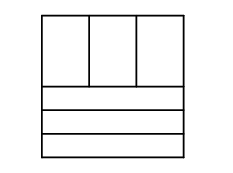Mathematical and Physical Journal
for High Schools
Issued by the MATFUND Foundation
 Already signed up? New to KöMaL?

#Problem K. 368. (February 2013)

K. 368. A square sheet of paper was cut into two equal parts along a line parallel to its side. Then one of the pieces was cut into three equal parts along lines parallel to its shorter side, and the other piece was cut into three equal parts along lines parallel to its longer side. The total perimeter of the resulting six sheets is 72 cm. What is the perimeter of the original square in centimetres?

(6 pont)

Deadline expired on March 11, 2013.

Sorry, the solution is available only in Hungarian. Google translation

Megoldás. Az eredeti négyzet oldala legyen 6 egységnyi. Az egyik féle kis lap kerülete 1+6+1+6=14 egységnyi, a másik féle kis lap kerülete pedig $\displaystyle 2+3+2+3=10$ egységnyi. Az összes kis lap kerülete így $\displaystyle 42+30=72$ egységnyi, azaz 1 egység 1 cm. Így az eredeti négyzet kerülete 24 cm.### Statistics:

 156 students sent a solution. 6 points: 74 students. 5 points: 39 students. 4 points: 7 students. 3 points: 4 students. 2 points: 5 students. 1 point: 2 students. 0 point: 24 students. Unfair, not evaluated: 1 solutions.

Problems in Mathematics of KöMaL, February 2013